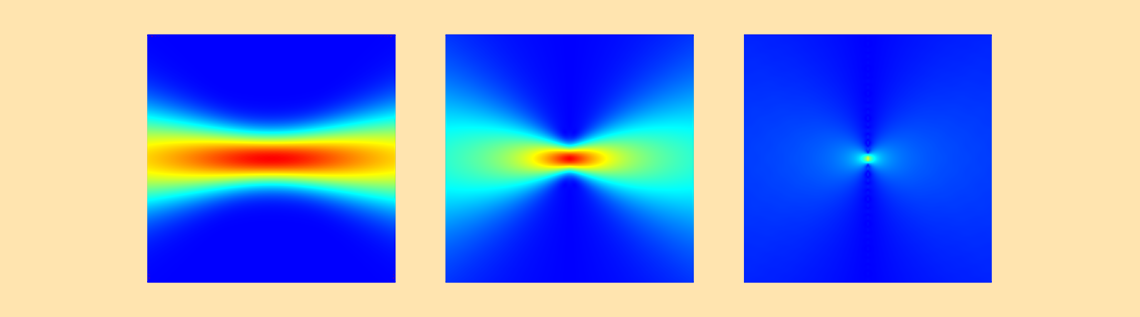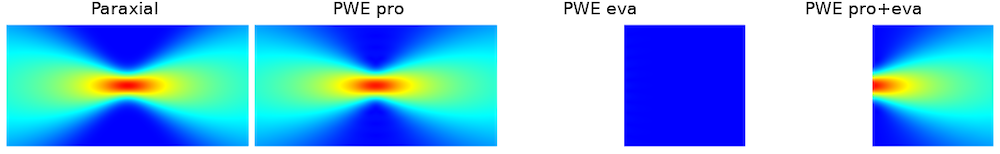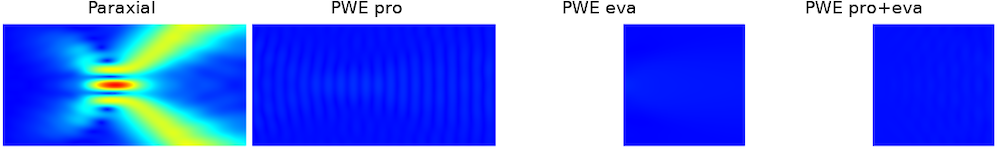# 非近轴高斯光束的倏逝分量

2020年 5月 12日

### 近轴和非近轴高斯光束简介

COMSOL 软件从 5.3a 版本开始引入了非近轴高斯光束背景场。对于非近轴情况，使用该公式比使用近轴高斯光束更好。非近轴高斯光束的公式由两部分组成：

1. 传播分量
2. 倏逝分量

### 角谱公式

E_x(x,y,z) = E_{x,0}\iint_{-\infty}^{\infty}A_x(k_x,k_y) {\rm exp}[i(k_x x +k_y y+k_z z)]dk_xdk_y,

E_y(x,y,z) = E_{y,0}\iint_{-\infty}^{\infty}A_y(k_x,k_y) {\rm exp}[i(k_x x +k_y y+k_z z)]dk_xdk_y,

E_z(x,y,z) = -\iint_{-\infty}^{\infty}\left [ \frac{k_x}{k_z} E_{x,0}A_x(k_x,k_y) + \frac{k_y}{k_z} E_{y,0}A_y(k_x,k_y) \right ] {\rm exp}[i(k_x x +k_y y+k_z z)]dk_xdk_y,

A_i(k_x,k_y) = \frac{w_0^2}{2\pi}{\rm exp}[-w_0^2(k_x^2+k_y^2)], \ i = x,y

### 在 COMSOL Multiphysics® 中模拟非近轴高斯光束

#### 混叠### 结束语

1. H. Kogelnik and T. Li, “Laser beams and resonators”, Applied Optics, vol. 5, no. 10, pp. 1550–1567, 1966.
2. “Gaussian beam”, Wikipediahttps://en.wikipedia.org/wiki/Gaussian_beam.
3. P.C. Chaumet, “Fully vectorial highly nonparaxial beam close to the waist”, JOSA A, vol. 23, no. 12, pp. 3197–3202, 2006.
4. R. Martinez-Herrero, P.M. Mejias, and A. Carnicer, “Evanescent field of vectorial highly non-paraxial beams”, Optics Express, vol. 16, no. 5, pp. 2845–2858, 2008.
5. P. Varga and P. Török, “The Gaussian wave solution of Maxwell’s equations and the validity of scalar wave approximation”, Optics Communications, vol. 152, no. 1–3, pp. 108–118, 1998.

#### 评论 (2)

##### 留言##### 禹昆 冀
2022-04-21##### 宇 念
2022-06-14 COMSOL 员工